Published: 07 January 2023

# Position control of electro-hydraulic servo system using active disturbance rejection control for upper-limb exoskeleton

Jing Tang1
Jiaxun Cao2
Minghu Wu3
Lun Zhao4
Fan Zhang5
1, 2, 3, 4, 5School of Electrical and Electronic Engineering, Hubei University of Technology, Wuhan, China
1, 3, 5Hubei Collaborative Innovation Center for High-efficiency Utilization of Solar Energy, Hubei University of Technology, Wuhan 430068, China
Corresponding Author:
Minghu Wu
Views 99

#### Abstract

This paper presents a two-degree-of-freedom(2-DOF) upper-limb exoskeleton actuated by electro-hydraulic servo system (EHSS), and position control based on active disturbance rejection control (ADRC) strategy. Proportional integral derivative (PID) controller is widely used in EHSS system because it is model free, and its parameters can be adjusted easily. However, the nonlinear dynamics of EHSS is subject to parameter variations and friction effects during operation. The trajectory tracking performance of this method is limited due to the uncertain model parameter and external disturbance of EHSS in exoskeleton. To actively compensate the total disturbance including the system uncertainty and external disturbance, and ensure the finite time convergence of disturbances, the ADRC controller is developed. The disturbances can be estimated by the extended state observer (ESO) and compensated during each sampling period in the ADRC method. The proposed control strategy not only satisfies the steady-state accuracy demands, but also effectively resists to the system uncertainties and the disturbance. To validate the feasibility of the proposed control strategy, the simulations were carried out. The numerical simulation results clearly indicate the superior performance of proposed ADRC method over the regular PID control approach.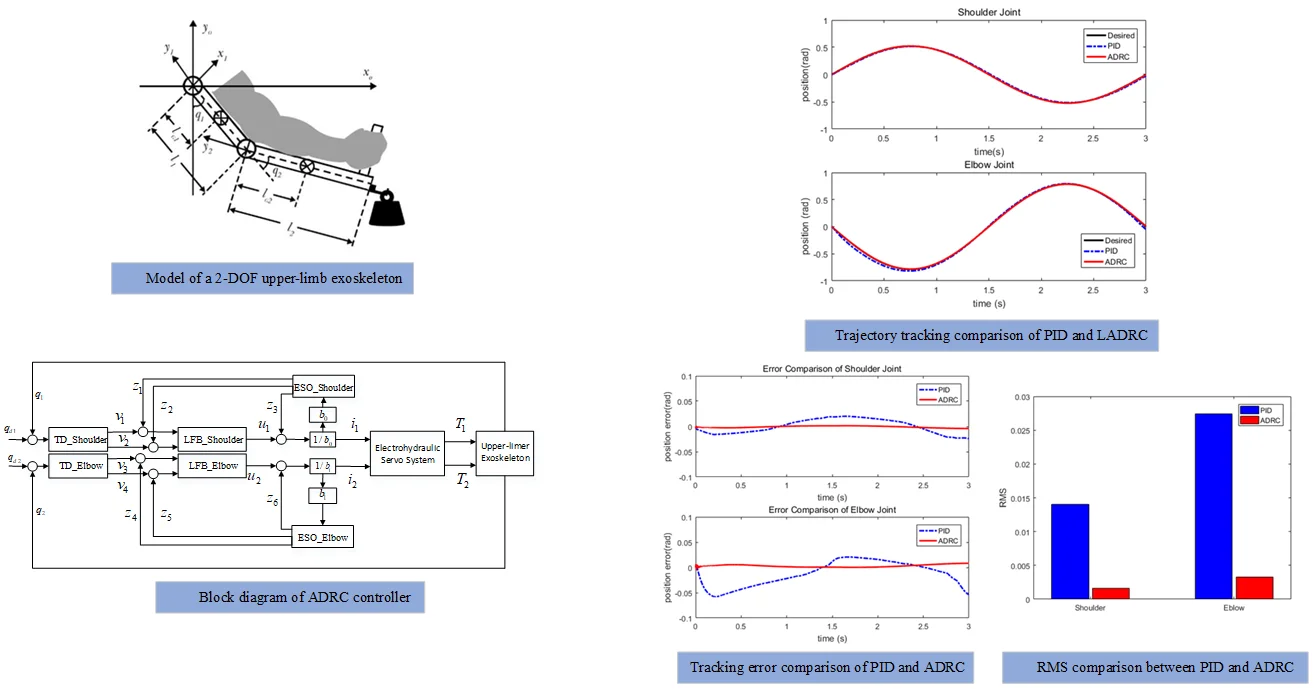#### Highlights

• The proposed control strategy not only satisfies the steady-state accuracy demands, but also effectively resists to the system uncertainties and the disturbance.
• The ADRC was proposed to improve the performance of the upper-limb exoskeleton through the disturbance estimating and finite-time convergence.
• The effectiveness of the proposed method is verified by numerical simulation through comparing with the PID and ADRC method.

## 1. Introduction

Upper-limb exoskeleton robots are generally applied in a variety of different fields, such as rehabilitation robots [1-3], assistive robots [4-6], human amplifiers [7, 8] and other uses. Robotic exoskeleton is the complex multi-input and multi-output (MIMO) with time varying dynamics and highly coupled nonlinear . Compared with industrial manipulators, exoskeletons suffer severe kinematic and dynamic uncertainties and external disturbances. Electro-hydraulic actuators have been used in exoskeleton in a wide number of applications by virtue of their high power to weight ratio and the ability to apply very large force and torque. However, the dynamics of hydraulic systems are highly nonlinear . Aside from the nonlinear of the electro-hydraulic actuators, they also have large extent of model uncertainties and uncertain nonlinearities such as the frictions. Moreover, the robotic exoskeleton and the actuators are coupled with each other, and there are uncertainties, un-modeled dynamics and disturbance . The design of upper-limb exoskeleton is one of the most challenging tasks to operate under various complex environmental conditions.

The conventional proportional integration derivative (PID) controller is widely used because of its simple control structure, ease of design, and low cost . However, the PID based controller are designed without considering the coupling, their performance is normally affected by the disturbance inputs and external disturbances. To attenuate disturbance and improve performance of the exoskeleton systems, several researchers have concentrated on exploring diverse control techniques, such as sliding mode control (SMC), and fuzzy logic control, active disturbance rejection control(ADRC) and so on. Teng et al., proposed a method combining PID control, sliding mode control, and fuzzy logic control, PD-based fuzzy sliding mode control, is developed to deal with unmodeled dynamics and external disturbances in the human-exoskeleton system . Sun et al., presented a reduced adaptive fuzzy system together with a compensation term . Compared to traditional approaches, the proposed fuzzy control approach can reduce possible chattering phenomena and achieve better control performance. Torabi. M et al., designed adaptive SMC scheme to provide robustness against disturbances with unknown bounds .

Apart from model-based controllers depend on accurate knowledge of the system, non-model based methods exist such as ADRC. To actively estimate and compensate for disturbance, some ADRC techniques are further studied. The original ADRC was first proposed by Han in 1998. As the core of the ADRC, the nonlinear extended state observer (ESO) can estimate and compensate for the total disturbance in the system to achieve stronger anti disturbance performance . The ADRC technique based on ESO, has been successfully used in robot application . Huang et al. presented an optimized linear active disturbance rejection controller to improve the response rapidity and anti-interference ability of a pneumatic servo system . Pawar S. N. et al. designed a modified reduced order ESO based ADRC to estimate the lumped disturbance actively as an extended state and compensate its effect by adding it to the control . As an alternative, the scheme avoids the complete knowledge of the dynamics, simplifying the model to a set of disturbed systems where the set of disturbances includes external disturbance inputs, internal perturbation and non-mode dynamics, which are on-line estimated for subsequent proper cancellation [20, 21]. Since the stability condition of the closed-loop system is expressed in the form of an explicit set of parameters, the tuning law of the ADRC can be intuitively obtained and described in engineering practice.

The goal is to design a controller for upper-limb exoskeleton actuated by hydraulic that can provide good position tracking to compensate for the robot’s dynamics and can provide comfortable human-machine interaction. The main contributions of this paper are listed as follows. First, the ADRC was proposed to improve the performance of the upper-limb exoskeleton through the disturbance estimating and finite-time convergence. Second, the effectiveness of the proposed method is verified by numerical simulation through comparing with the PID and ADRC method. This paper is organized as follows. The next section presents the 2-DOF upper-limb exoskeleton kinematic model and the EHSS model. While the ADRC method for position control is presented in Section 3. To demonstrate the performance of the proposed method, the simulation results are presented in Section 4. The main content is summarized and key point of future work are discussed in the final section.

## 2. System modeling

### 2.1. Modeling of upper-limb exoskeleton

The exoskeleton is a typical human-robot collaboration system. The control systems of exoskeleton are different from the traditional robots, because the human operator is not the commander of the system, but also a part of the control loop. Hence, the human operator mainly makes the decisions, then the exoskeleton implements the tasks. A model of a 2-DOF upper-limb exoskeleton consisting of the load, the exoskeleton and an operator is shown in Fig. 1. The upper-limb exoskeleton has 2 DOFs, with the shoulder joint and elbow joint actuated and capable of following the rotations of the corresponding joints of the operator.

Without loss of generality, the dynamic equation of the upper-limb exoskeleton robot can be express as follows:

1
$\mathbf{T}+{\mathbf{T}}_{\mathbf{d}}=\left(\mathbf{M}\left(q\right)+\mathbf{\Delta }\mathbf{M}\left(q\right)\right)\stackrel{¨}{\mathbf{q}}+\left(\mathbf{C}\left(q,\stackrel{˙}{q}\right)+\mathbf{\Delta }\mathbf{C}\left(q,\stackrel{˙}{q}\right)\right)+\left(\mathbf{G}\left(q\right)+\mathbf{\Delta }\mathbf{G}\left(q\right)\right),$

where $\mathbf{T}=\left[{T}_{1},{T}_{2}{\right]}^{T}\in {R}^{2×1}$ is a vector of actuator joint torque; $\mathbf{q}=\left[{q}_{1,}{q}_{2}{\right]}^{T}\in {R}^{2×1}$ is a vector of exoskeleton angles for revolute joints; $\stackrel{˙}{\mathbf{q}}$ and $\stackrel{¨}{\mathbf{q}}$ are the joint velocities and accelerations respectively. ${\mathbf{T}}_{d}$ is the total disturbances of the system. Meanwhile, $\mathbf{M}\left(q\right)$ is the inertia matrix, $\mathbf{C}\left(q,\stackrel{˙}{q}\right)$ is the centripetal matrix, and $\mathbf{G}\left(q\right)$ is the gravity torque, respectively. $\mathbf{\Delta }\mathbf{M}\left(q\right)$, $\mathbf{\Delta }\mathbf{C}\left(q,\stackrel{˙}{q}\right)$ and $\mathbf{\Delta }\mathbf{G}\left(q\right)$ denote the uncertainty of the robot system. The specific expression of the matrices $\mathbf{M}$, $\mathbf{C}$ and $\mathbf{G}$ can be described as:

2
$\mathbf{M}=\left[\begin{array}{ll}{M}_{11}& {M}_{12}\\ {M}_{21}& {M}_{22}\end{array}\right],\mathbf{}\mathbf{}\mathbf{}\mathbf{}\mathbf{}\mathbf{C}=\left[\begin{array}{l}{C}_{1}\\ {C}_{2}\end{array}\right],\mathbf{}\mathbf{}\mathbf{}\mathbf{}\mathbf{}\mathbf{G}=\left[\begin{array}{l}{G}_{1}\\ {G}_{2}\end{array}\right],$

where

$\left\{\begin{array}{l}{M}_{11}={I}_{1}+{I}_{2}+{m}_{1}{{l}_{G1}}^{2}+{m}_{2}\left({{l}_{1}}^{2}+{{l}_{G2}}^{2}\right)+2{m}_{2}{l}_{1}{l}_{G2}\mathrm{c}\mathrm{o}\mathrm{s}{q}_{2},\\ {M}_{12}={I}_{2}+{m}_{2}{{l}_{G2}}^{2}+{m}_{2}{l}_{1}{l}_{G2}\mathrm{c}\mathrm{o}\mathrm{s}{q}_{2},\\ {M}_{21}={I}_{2}+{m}_{2}{{l}_{G2}}^{2}+{m}_{2}{l}_{1}{l}_{G2}\mathrm{c}\mathrm{o}\mathrm{s}{q}_{2},\\ {M}_{22}={I}_{2}+{m}_{2}{{l}_{G2}}^{2},\end{array}\right\$
$\left\{\begin{array}{l}{C}_{1}=-\left({m}_{2}+{m}_{l}\right){l}_{1}{l}_{G2}\left(2{q}_{1}{q}_{2}+{\stackrel{˙}{q}}_{2}^{2}\right)\mathrm{s}\mathrm{i}\mathrm{n}\left({q}_{2}\right)-\left({m}_{2}+{m}_{l}\right){l}_{1}{l}_{G2}{\stackrel{˙}{q}}_{1}^{2}\mathrm{s}\mathrm{i}\mathrm{n}\left({q}_{2}\right),\\ {C}_{2}=\left({m}_{2}+{m}_{l}\right){l}_{1}{l}_{G2}{\stackrel{˙}{q}}_{1}^{2}\mathrm{s}\mathrm{i}\mathrm{n}\left({q}_{2}\right),\end{array}\right\$
$\left\{\begin{array}{l}{G}_{1}=\left({m}_{2}+{m}_{l}\right)g{l}_{G2}\mathrm{s}\mathrm{i}\mathrm{n}\left({q}_{1}+{q}_{2}\right)+{m}_{1}g{l}_{G1}\mathrm{s}\mathrm{i}\mathrm{n}\left({q}_{1}\right)+\left({m}_{2}+{m}_{l}\right)g{l}_{1}\mathrm{s}\mathrm{i}\mathrm{n}\left({q}_{1}\right),\\ {G}_{2}=\left({m}_{2}+{m}_{l}\right)g{l}_{G2}\mathrm{s}\mathrm{i}\mathrm{n}\left({q}_{1}+{q}_{2}\right),\end{array}\right\$

where ${m}_{1}$ is the mass of the robotic upper arm, ${m}_{2}$ is the mass of the robotic forearm, ${l}_{1}$ is the length of the robotic upper arm, ${m}_{l}$ is the mass of external load, ${l}_{2}$ is the length of the robotic forearm, ${l}_{G1}$ is the position of the center of the robotic upper arm, ${l}_{G2}$ is the position of the center of the robotic forearm, ${I}_{1}$ is the inertia of the robotic upper arm and ${I}_{2}$ is the inertia of the robotic forearm.

Fig. 1Model of a 2-DOF upper-limb exoskeleton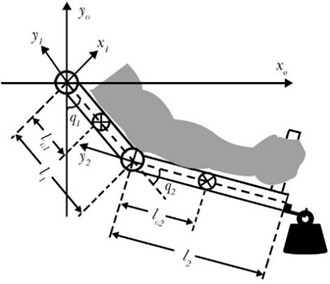Based on the dynamics model of robotic exoskeleton shown by Eq. (1), The system should be decoupled, and matrix $\mathbf{M}\left(q\right)+\mathbf{\Delta }\mathbf{M}\left(q\right)$ can be approximated as $\mathbf{M}\left(q\right)$. Assumption:

3
$\mathbf{\lambda }\left(q,\stackrel{˙}{q},t\right)={\mathbf{T}}_{d}-\mathbf{\Delta }\mathbf{C}\left(q,\stackrel{˙}{q}\right)-\mathbf{\Delta }\mathbf{G}\left(q\right).$

The mathematical model of robotic exoskeleton can be expressed as below:

4
$\mathbf{M}\left(q\right)\stackrel{¨}{\mathbf{q}}+\mathbf{C}\left(q,\stackrel{˙}{q}\right)+\mathbf{G}\left(q\right)=\mathbf{T}+\mathbf{\lambda }\left(q,\stackrel{˙}{q},t\right).$

The inertia matrix is symmetric positive definite, Eq. (4) can be rewritten as:

5
$\stackrel{¨}{\mathbf{q}}=\mathbf{\tau }+\mathbf{f},$

where$\mathbf{\tau }={\mathbf{M}}^{-1}\left(q\right)\mathbf{T}$, $\mathbf{f}={\mathbf{M}}^{-1}\left(q\right)\left(\mathbf{\lambda }\left(q,\stackrel{˙}{q},t\right)-\mathbf{C}\left(q,\stackrel{˙}{q}\right)-\mathbf{G}\left(q\right)\right)$. $\mathbf{f}=\left[{f}_{1},{f}_{2}{\right]}^{T}$ is the total disturbance of the system, including system error, external disturbance and couple term. $\stackrel{¨}{\mathbf{q}}={\left[{\stackrel{¨}{q}}_{1},{\stackrel{¨}{q}}_{2}\right]}^{T}$ is the acceleration of the revolute joints.

### 2.2. Modeling of the electro-hydraulic servo system

The structure of EHSS is shown in Fig. 2. In many EHSS applications, valve dynamics is much faster than other parts of the system. Therefore, valve dynamics can be neglected, we assumed that the servo valve as a proportional block, by following approximation:

6
${x}_{v}={k}_{c}i,$

where ${k}_{c}$ is a positive electrical constant, and $i$ is the input current.

Fig. 2The structure of EHSS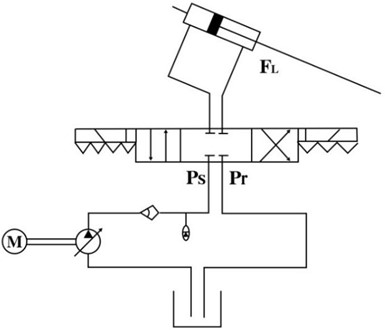By applying the continuity law to each actuator chamber, the external leakage of EHSS is extremely small and can be neglected. The flow equation of actuator’s valve is described as:

7
$\left\{\begin{array}{l}{Q}_{1}={C}_{d}w\sqrt{\frac{2}{\rho }}{x}_{v}\left[s\left({x}_{v}\right)\sqrt{{P}_{s}-{P}_{1}}+s\left(-{x}_{v}\right)\sqrt{{P}_{1}-{P}_{r}}\right],\\ {Q}_{2}={C}_{d}w\sqrt{\frac{2}{\rho }}{x}_{v}\left[s\left({x}_{v}\right)\sqrt{{P}_{2}-{P}_{r}}+s\left(-{x}_{v}\right)\sqrt{{P}_{s}-{P}_{1}}\right],\end{array}\right\$

where ${Q}_{1}$ is the supplied flow rate to the forward chamber; ${Q}_{2}$ is return flow rate of the return chamber. ${C}_{d}$ is the discharge coefficient; $w$ is the spool valve area gradient; $\rho$ is the density of hydraulic oil; ${P}_{s}$ is the supply pressure of the fluid; ${P}_{r}$ is the return pressure; ${P}_{1}$ is the head-side pressure; ${P}_{2}$ is the rod-side pressure; $S\left({x}_{v}\right)$ is defined as:

8
$s\left({x}_{v}\right)=\left\{\begin{array}{l}1,\begin{array}{ll}& \end{array}{x}_{v}\ge 0,\\ 0,\begin{array}{ll}& \end{array}{x}_{v}<0.\end{array}\right\$

The pressure dynamics in actuator chambers can be described as:

9
$\left\{\begin{array}{l}{\stackrel{˙}{P}}_{1}=\frac{\beta }{{V}_{1}}\left[-{A}_{p1}{v}_{p}-{C}_{t}{P}_{L}+{Q}_{1}\right],\\ {\stackrel{˙}{P}}_{2}=\frac{\beta }{{V}_{2}}\left[{A}_{p1}{v}_{p}+{C}_{t}{P}_{L}-{Q}_{2}\right],\end{array}\right\$

where ${V}_{1}$, ${V}_{2}$ are the control volumes of the actuator chambers; $\beta$ is the effective bulk modulus of the system;${C}_{t}$ is the coefficient of the total internal leakage of the actuator due to the pressure; ${P}_{L}={P}_{1}-{P}_{2}$ is the load pressure of the dynamic actuator. The actuated force can be express as:

10
$\left\{\begin{array}{l}{F}_{L}={P}_{1}{A}_{p1}-{P}_{2}{A}_{p2},\\ {A}_{p1}=\frac{\pi {D}_{1}^{2}}{4},\\ {A}_{p2}=\frac{\pi \left({D}_{1}^{2}-{D}_{2}^{2}\right)}{4},\end{array}\right\$

where ${A}_{p1}$ is the head-side area; ${A}_{p2}$ is the rod-side area. ${D}_{1}$ is the bore diameter; ${D}_{2}$ is the rod diameter. As the rod diameter is small compared to the bore diameter, the ${A}_{p2}$ can be simplified as:

11
${A}_{p2}=\frac{\pi \left({D}_{1}^{2}-{D}_{2}^{2}\right)}{4}\approx \frac{\pi {D}_{1}^{2}}{4}={A}_{p1}.$

The EHSS driving torque $T$ can be express as:

12
$T=\left({P}_{1}-{P}_{2}\right){A}_{p1}H,$

where $H$ is arm of torque.

For the 2-DOF upper-limb exoskeleton, two sets of EHSS generate the force to drive the robotic shoulder and arm respectively:

13
$\mathbf{T}=\left[\begin{array}{l}{T}_{1}\\ {T}_{2}\end{array}\right]=\left[\begin{array}{l}\left({P}_{11}-{P}_{21}\right){A}_{p1}{H}_{1}\\ \left({P}_{12}-{P}_{22}\right){A}_{p1}{H}_{2}\end{array}\right],$

where ${T}_{1}$ and ${T}_{2}$ are the actuator torques of shoulder joint and elbow joint. ${P}_{11}$ and ${P}_{21}$ are the head-side pressure and rod-side pressure of shoulder joint. ${P}_{12}$ and ${P}_{22}$ are the head-side pressure and rod-side pressure of elbow joint. ${H}_{1}$ and ${H}_{2}$ are the arms of force for the robotic shoulder and elbow. Based on the geometry of robotic exoskeleton, the ${H}_{1}$ and ${H}_{2}$ can be calculated as:

14
$\left\{\begin{array}{l}{H}_{1}=\frac{ab\mathrm{s}\mathrm{i}\mathrm{n}\left({q}_{1}\right)}{\sqrt{{a}^{2}+{b}^{2}-2ab\mathrm{s}\mathrm{i}\mathrm{n}\left({q}_{1}\right)}},\\ {H}_{2}=\frac{-\left({l}_{1}-c\right)d\mathrm{c}\mathrm{o}\mathrm{s}\left({q}_{2}\right)}{\sqrt{\left({l}_{1}-c{\right)}^{2}+{d}^{2}+2\left({l}_{1}-c\right)d\mathrm{c}\mathrm{o}\mathrm{s}\left({q}_{2}\right)}},\end{array}\right\$

where $a$, $b$, $c$ and $d$ are the geometric length of the upper limb exoskeleton. Fig. 3 provides the design geometry of the exoskeleton hydraulic cylinder.

The parameters of Electro-hydraulic Servo system are used in Table 1.

## 3. Control strategy design for upper-limb exoskeleton

The purpose of our upper-limb exoskeleton design is to evaluate the position tracking performance. To implement this goal, the desired position should be acquired. In this system, the desired angles for revolute joints for the test purposes are sinusoidal signals at a sampling frequency of $f=$1000 Hz:

15
$\left\{\begin{array}{l}{q}_{d1}=\frac{\pi }{6}\mathrm{s}\mathrm{i}\mathrm{n}\left(2\pi ft\right),\\ {q}_{d2}=\frac{\pi }{4}\mathrm{s}\mathrm{i}\mathrm{n}\left(2\pi ft\right),\end{array}\right\\begin{array}{ll}& 0\le t\le 3.\end{array}$

Fig. 3The design geometry of the exoskeleton hydraulic cylinder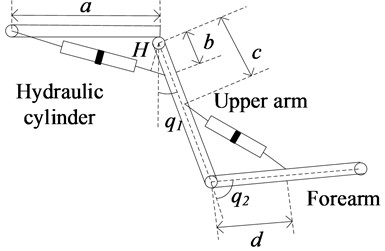Table 1Parameters of EHSS

 Parameter Representation Value ${A}_{p1}$ Head-side area 1.77×10-4 m2 ${A}_{p2}$ Rod-side area 1.57×10-4 m2 ${D}_{1}$ Bore diameter 0.015 m2 ${D}_{2}$ Rod diameter 0.0075 m2 ${P}_{r}$ Return pressure 5×104 Pa ${P}_{s}$ Supply pressure of the fluid 2×106 Pa $\mathrm{\rho }$ Density of hydraulic oil 830 kg m3 $\mathrm{w}$ Spool valve area gradient 9.59×10-3 m2 $\mathrm{\beta }$ Effective bulk modulus of the system 2×107 Pa ${C}_{d}$ Discharge coefficient 0.61 ${C}_{t}$ Coefficient of the total internal leakage 8×10-12 M5N-1s-1 ${k}_{c}$ Positive electrical constant 1.38×10-4 m3s-1Pa-1 ${V}_{1}$ Volume of the actuator chamber 1.4×10-4 m3 ${V}_{2}$ Volume of the actuator chamber 1.4×10-4 m3 ${l}_{1}$ Length of the robotic upper arm 0.45 m ${l}_{2}$ Length of the robotic forearm 0.5 m

### 3.1. Design PID controller for upper-limb exoskeleton

The conventional PID controller is widely adopt due to its simple structure and its gains can be easily tuned. The fixed PID controller of the hydraulically actuated exoskeleton system is shown in Fig. 4, and can be constructed as follows:

16
$\left\{\begin{array}{l}{i}_{1}\left(t\right)={K}_{p1}{e}_{1}\left(t\right)+{K}_{i1}{{e}_{1}}^{-1}\left(t\right)+{K}_{d1}{{e}_{1}}^{\text{'}}\left(t\right),\\ {i}_{2}\left(t\right)={K}_{p2}{e}_{2}\left(t\right)+{K}_{i2}{{e}_{2}}^{-1}\left(t\right)+{K}_{d2}{{e}_{2}}^{\text{'}}\left(t\right),\end{array}\right\$

where ${K}_{p1}$(${K}_{p2}$), ${K}_{i1}$(${K}_{i2}$) and ${K}_{d1}$(${K}_{d2}$) are proportional, integration and differential gains respectively. Moreover, ${e}_{1}\left(t\right)={q}_{d1}\left(t\right)-{q}_{1}\left(t\right)$ and ${e}_{2}\left(t\right)={q}_{d2}\left(t\right)-{q}_{2}\left(t\right)$ are the errors between the desired positions and actual positions.

Fig. 4Block diagram of PID controller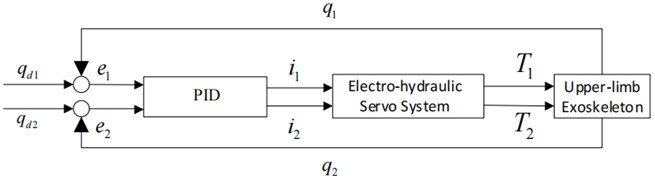### 3.2. Design ADRC for upper-limb exoskeleton

The ADRC method adopt nonlinear function, resulting in difficult analysis and parameter setting complex. Therefore, ADRC is designed based on the dynamic of robotic exoskeleton. In this paper, the dynamics model of the exoskeleton leg is multi-input and multi-output (MIMO) system. The Block diagram of ADRC controller is shown in Fig. 5. After system is decoupled, ADRC can be designed to control each joint respectively. Eq. (5) can be split into two independent equations for the shoulder and elbow joint as follow:

17
$\left\{\begin{array}{l}{\stackrel{¨}{q}}_{1}={\tau }_{1}+{f}_{1},\\ {\stackrel{¨}{q}}_{2}={\tau }_{2}+{f}_{2}.\end{array}\right\$

The ADRC controller consist three main parts, i.e., the tracking differentiator (TD) to provide derivative of the input signal, the extend state observer (ESO) to estimate the states and the disturbances and the nonlinear feedback to generate the control signal. TD is used to generate the arranged transition process and extract the input signal’s differential of each order. The tracking differentiator is designed in the form of:

18
$\left\{\begin{array}{l}{\stackrel{˙}{v}}_{1}={v}_{2},\\ {\stackrel{˙}{v}}_{2}={k}_{1}\left({v}_{1}-{q}_{d1}\right)-{k}_{2}{v}_{2},\end{array}\right\\left\{\begin{array}{l}{\stackrel{˙}{v}}_{3}={v}_{4},\\ {\stackrel{˙}{v}}_{4}={k}_{1}\left({v}_{3}-{q}_{d2}\right)-{k}_{2}{v}_{4},\end{array}\right\$

where ${q}_{d1}$ and ${q}_{d2}$ are desired angles, ${k}_{1}$ and ${k}_{2}$ are the observer gains to ensure convergence of the error. ESO is designed to estimate and compensation total disturbances of hydraulically actuated exoskeleton system. Two extended state observers can be obtained as follows:

19
$\left\{\begin{array}{l}{\stackrel{˙}{z}}_{1}={z}_{2}+{\beta }_{11}{e}_{1},\\ {\stackrel{˙}{z}}_{2}={z}_{3}+{\beta }_{12}{e}_{1}+{b}_{0}{i}_{1},\\ {\stackrel{˙}{z}}_{3}={\beta }_{13}{e}_{1},\end{array}\right\$
$\left\{\begin{array}{l}{\stackrel{˙}{z}}_{4}={z}_{5}+{\beta }_{21}{e}_{2},\\ {\stackrel{˙}{z}}_{5}={z}_{6}+{\beta }_{22}{e}_{2}+{b}_{1}{i}_{2},\\ {\stackrel{˙}{z}}_{6}={\beta }_{23}{e}_{2},\end{array}\right\$

where ${\beta }_{11}$, ${\beta }_{12}$, ${\beta }_{13}$ and ${\beta }_{21}$, ${\beta }_{22}$, ${\beta }_{23}$ are the observer gains. The observations error are defined as ${e}_{1}={z}_{1}-{q}_{1}$, ${e}_{2}={z}_{4}-{q}_{2}$. For the purpose of tuning simplification, all the observer poles are placed at $-{\omega }_{0}$. The observer gains can be determined according to the characteristic polynomial :

20
$\begin{array}{l}{s}^{3}+{\beta }_{11}{s}^{2}+{\beta }_{12}s+{\beta }_{13}=\left(s+{w}_{0}{\right)}^{3},\\ {s}^{3}+{\beta }_{21}{s}^{2}+{\beta }_{22}s+{\beta }_{23}=\left(s+{w}_{0}{\right)}^{3},\end{array}$

where ${\omega }_{0}$ is the bandwidth of observer and the gain vector can be expressed as follows: ${\beta }_{11}=3{\omega }_{0}$, ${\beta }_{12}=3{{\omega }_{0}}^{2}$, ${\beta }_{13}={\omega }_{0}^{3}$ and ${\beta }_{21}=3{\omega }_{0}$, ${\beta }_{22}=3{{\omega }_{0}}^{2}$, ${\beta }_{23}={\omega }_{0}^{3}$. In this paper, the bandwidth of two observers is defined as the same values of $-{\omega }_{0}$. The bandwidth of feedback controller is defined as ${\omega }_{c}=\frac{1}{3}{\omega }_{0}$. Finally, the feedback control law in the proposed control can be written as follows:

21
$\left\{\begin{array}{l}{u}_{1}={k}_{p1}\left({z}_{1}-{v}_{1}\right)+{k}_{d1}\left({z}_{2}-{v}_{2}\right),\\ {i}_{1}={u}_{1}-\frac{{z}_{3}}{{b}_{0}},\begin{array}{lll}& & \end{array}\begin{array}{lll}& & \end{array}\end{array}\right\$
$\left\{\begin{array}{l}{u}_{2}={k}_{p2}\left({z}_{4}-{v}_{3}\right)+{k}_{d2}\left({z}_{5}-{v}_{4}\right),\\ {i}_{2}={u}_{2}-\frac{{z}_{6}}{{b}_{1}}\begin{array}{lll},& & \end{array}\begin{array}{lll}& & \end{array}\end{array}\right\$

where ${k}_{p1}$, ${k}_{p2}$ and ${k}_{d1}$, ${k}_{d2}$ are the proportional and differential gains, respectively.

Fig. 5Block diagram of ADRC controller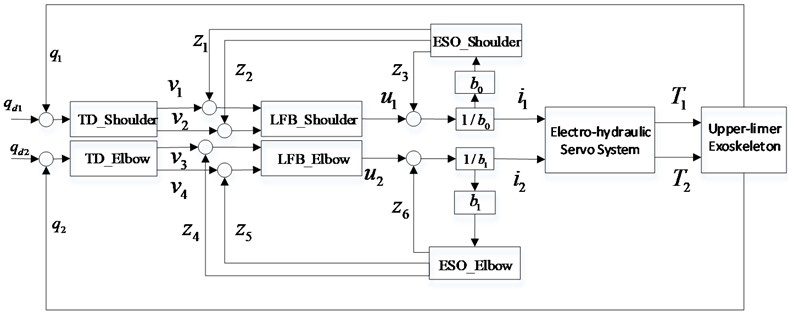## 4. Simulation results

### 4.1. Selection of system parameters

The objectives of this section are to compare the tracking performance of PID control approach and the ADRC strategy. There are two ESOs to estimate existing disturbance for the shoulder joint and the elbow joint respectively. The parameters of the EHSS and the upper-limb exoskeleton are listed in Table 1. Two simulations are carried out, in MATLAB, by using PID control strategy and the ADRC. The parameters of ADRC are shown in Table 2, ${b}_{0}$ and ${b}_{1}$ are the amplification coefficients of ${i}_{1}$ and ${i}_{2}$. ${w}_{0}$ is the bandwidth of observer, and the selection of its value affect the tracking effect.

 Symbol Value Symbol Value ${b}_{0}$ 1 ${b}_{1}$ 4 ${w}_{0}$ 1200 ${\beta }_{21}$ 3600 ${\beta }_{11}$ 3600 ${\beta }_{22}$ 4.32×106 ${\beta }_{12}$ 4.32×106 ${\beta }_{23}$ 1.728×109 ${\beta }_{13}$ 1.728×109 ${k}_{p2}$ 160000 ${k}_{p1}$ 160000 ${k}_{d2}$ 800 ${k}_{d1}$ 800

Fig. 6 shows the tracking error of each joint with different ${w}_{0}$ in proposed ADRC. As seen in the figure, the error decrease as the value of ${w}_{0}$ increases, the larger the value of ${w}_{0}$ is, the faster the errors converge.

Fig. 6Tracking error with different w0 in proposed ADRC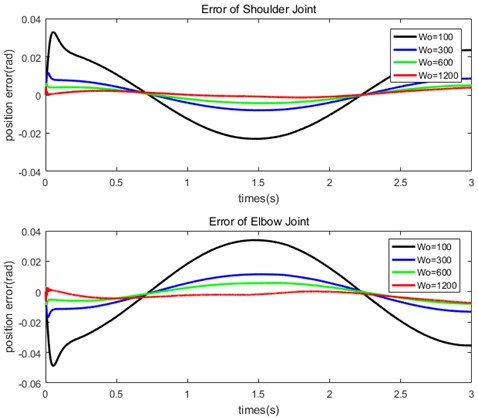### 4.2. Comparisons of the conventional PID controller and ADRC controller

The system simulations are carried out, in MATLAB (Version R2016a), by using PID control strategy and ADRC approach presented in respectively. The parameters of the controllers are chosen using Ziegler and Nichols method . Then, the optimum parameters for the PID controller can be provided as ${K}_{p1}=$ 240, ${K}_{i1}=$ 40, ${K}_{d1}=$ 180 and ${K}_{p2}=$ 200, ${K}_{i2}=$ 80, ${K}_{d2}=$ 120. The external disturbance is generated by a sinusoidal signal as follows:

22
${f}_{1}={f}_{2}=0.01\mathrm{s}\mathrm{i}\mathrm{n}\left(10\pi t\right).$

Fig. 7Trajectory tracking comparison of PID and LADRC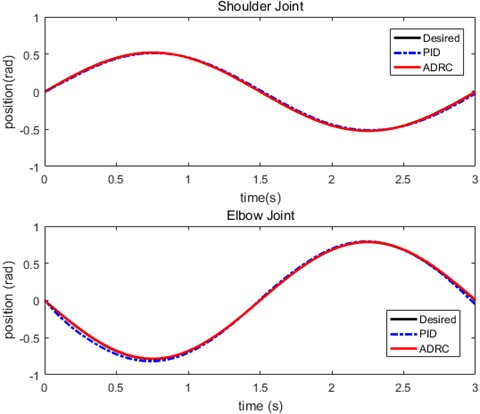Performances of the conventional PD controller and ADRC method for position trajectory control are compared next. Figs. 7, 8 show trajectory tracking, position errors for each joint. As shown in the Fig. 7, The trajectories produced by the PID controller is depicted in red color, then ADRC is depicted in blue color, and the desired angle is depicted in black color. The performance of ADRC method is better compared to the conventional PID control. Fig. 8 shows the trajectory tracking error of the shoulder joint and the elbow joint respectively. It is seen that the deviations occur from the desired trajectory and ADRC controller produces better results than the conventional PID controller. The ADRC controller possess 3 ms lag, which is smaller than 11ms lag for the PID controller.

Fig. 8Tracking error comparison of PID and ADRC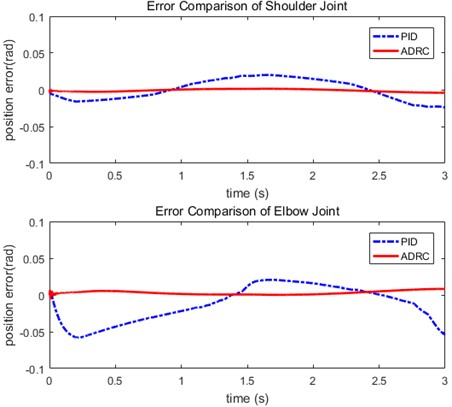To evaluate the performance of the general PID control approach and the proposed method, the tracking errors are compared. The tracking performances are indicated by root-mean-square (RMS) error :

23
$RMS=\sqrt{\frac{1}{n}\sum _{1}^{n}\left(q-{q}_{d}{\right)}^{2}}.$

Fig. 9RMS comparison between PID and ADRC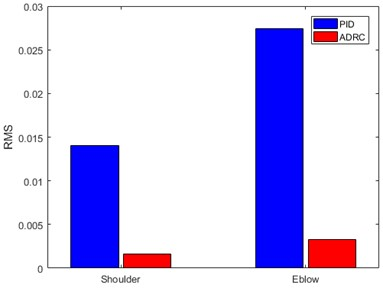Fig. 9 provides the RMS errors of the human-machine position. From Fig. 9, both of the tracking errors of shoulder joint and elbow joint under the ADRC approach are smaller than those under the conventional PID control strategy. It is obviously to see that the proposed ADRC method is better than PID control with its robustness to external disturbance or un-modeled system dynamic, and it will be easy to implement.

## 5. Conclusions

The ADRC strategy for 2-DOF upper-limb exoskeleton, under the actuation of hydraulic, was explored in this paper. Considering the unavailability of precise model parameters and the uncertain disturbances in upper-limb exoskeleton actuated by EHSS. The proposed control strategies not only reduce the influence of unknown disturbances and eliminates the dependence on accurate model parameters, but also improve the tracking accuracy of the exoskeleton system and make the error converge quickly. The simulation results validate the effectiveness of the proposed method. The limitation of our approach is tuning the ADRC parameters automatically. In the future, the combination of ADRC with other model free tuning techniques will be investigated to reduce this limitation. It is proposed for the further development to investigate the robustness of ADRC for the external load uncertainty and motion velocity change. Moreover, Future work will include applying different types of other path tracking trajectories.

#### Acknowledgements

This work was supported by Key Research and Development Plan of Hubei Province (2021BGD013), Hubei University of Technology Ph.D. Research Startup Fund Project (BSQD2020014) and Science and Technology Research Program of Hubei Provincial Department of Education (T201805).